Image Processing Toolbox User's GuideUsing the Radon Transform to Detect Lines

The Radon transform is closely related to a common computer vision operation known as the Hough transform. You can use the radon function to implement a form of the Hough transform used to detect straight lines. The steps are

1. Compute a binary edge image using the edge function.
• I = fitsread('solarspectra.fts');
I = mat2gray(I);
BW = edge(I);
imshow(I), figure, imshow(BW)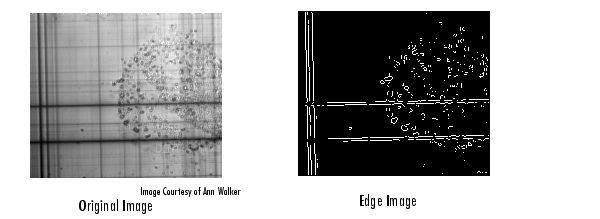2. Compute the Radon transform of the edge image.
• theta = 0:179;
figure, imagesc(theta, xp, R); colormap(hot);
xlabel('\theta (degrees)'); ylabel('x\prime');
title('R_{\theta} (x\prime)');
colorbar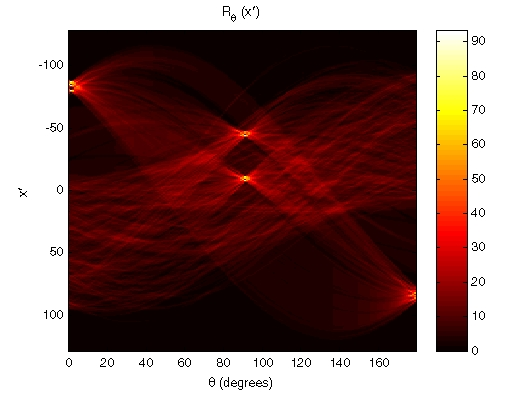Radon Transform of an Edge Image

1. Find the locations of strong peaks in the Radon transform matrix. The locations of these peaks correspond to the locations of straight lines in the original image.

In the following figure, the strongest peaks in R correspond to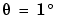and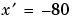. The line perpendicular to that angle and located atis shown below, superimposed in red on the original image. The Radon transform geometry is shown in black. Notice that the other strong lines parallel to the red line also appear as peaks atin the transform. Also, the lines perpendicular to this line appear as peaks at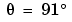.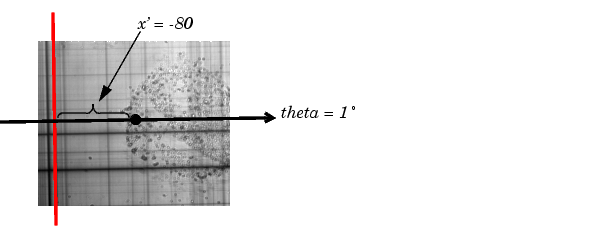Radon Transform Geometry and the Strongest Peak (Red)Viewing the Radon Transform as an Image Inverse Radon Transform